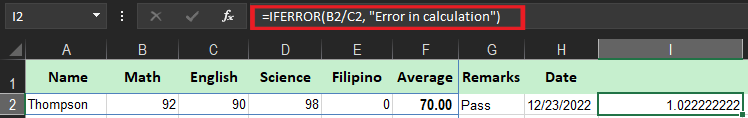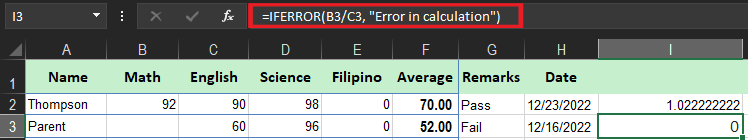# IFERROR Function In Excel How To Use It With Examples

In this tutorial, you will know the IFerror function in excel and how to use it. Particularly, you will learn how this function catch up with errors and replace them with a blank cell, another value, or a custom message.

Thus, you will discover how to use this function with Vlookup and Index match, and how this function differs from IF ISERROR and IFNA.

In this tutorial, we won’t be looking at how to return errors in Excel. Instead, you will know how to prevent errors in order to keep the worksheet clean and the formulas as well.

## what is the iferror function in excel

The IFERROR function is the Excel function which is designed to manage and prevent errors in the formulas and functions calculations. Precisely, this function is tasked to check the formula and if it determines as an error the function will return the values you specify otherwise it will return the output of the formula.

Furthermore, The IFFERROR function is a friendly function which returns a result and message in approachable when error is caught and detected.

The syntax of the Excel IFERROR function is as follows:

``IFERROR(value, value_if_error)``

Where the arguments explain:

• Value – A required argument, and the value to check for errors, thus it can be a cell reference, formula, expression
• Value_if_error – A required argument and a return value when an error is found. It can be an empty string (blank cell), text message, numeric value, another formula or calculation.

Here are the forms you want to handle errors:

## If error, then blank

Supply an empty string (“) to the value_if_error argument to return a blank cell if an error is found:

``=IFERROR(A2/B2, "")``

## If error, then show a message

You can also display your own message instead of Excel’s standard error notation:

``=IFERROR(A2/B2, "Error in calculation")``

## how to use the iferror function in excel

Now we will provide examples that explain how to use IFERROR function, along with other functions to perform the tough tasks.

Time needed: 3 minutes

Specifically, array formulas are intended to execute multiple functions on a single formula. If you provide the array formula or expression that results in an array in the value argument of the IFERROR function, it’d return an array of values for each cell in the specified range.

The below example shows the details.

1. =IFERROR(B2/C2, “Error in calculation”)

It checks if the formula has an error in the first argument otherwise it will return the results of the formula.2. =IFERROR(B3/C3, “Error in calculation”)

It checks if the formula has an error in the first argument otherwise it will return the results of the formula.3. =IFERROR(B4/C4, “Error in calculation”)

It checks if the formula has an error in the first argument and finds the zero value hence returning an error message.## IFERROR function in excel vlookup

You can also use the IFERROR function to catch the #N/A error thrown by VLOOKUP when a lookup value isn’t found. The syntax looks like this:

``=IFERROR(VLOOKUP(value,data,column,0),"Invalid Entry")``

This syntax explains when VLOOKUP returns a result,  IFERROR functions return that result. Whereas if VLOOKUP returns #N/A error because a lookup value isn’t found, IFERROR returns “Invalid Entry”.

### IFERROR or IFNA

So IFNA function is introduced in Excel 2013 it is one of the functions which checks a formula for errors. Its syntax is similar to that of IFERROR:

``IFNA(value, value_if_na)``

Apparently, even iferror function is useful it is a blunt tool where it will hold many errors. Like in certain instances where there is a typo in a formula. Thus Excel will return #NAME? error, but IFERROR will suppress the error and return the alternative result.

This can cover an important problem. In numerous circumstances, it is reasonable to use the IFNA function, which only traps the #N/A error.

## Other error functions

Excel provides a number of error-related functions, each with a different behavior:

• The ERROR.TYPE function returns the numeric code for a given error.
• The IFERROR function traps errors and supplies an alternative result.
• The IFNA function traps #N/A errors and gives an alternative result.
• The ISERR function returns TRUE for any error type except the #N/A error.
• The ISERROR function returns TRUE for any error.
• The ISNA function returns TRUE for #N/A errors only.

## Conclusion

In conclusion, we discussed IFerror Function In Excel How To Use It With Examples this will help you in handling errors in your worksheet.

• If the cell is empty. it is determined as an empty string(“”) and not an error.
• No message will be displayed if the error is detected if the value_if_error is supplied as an empty string (“”),
• You can use IFNA In Excel 2013+ to trap and handle #N/A errors particularly.